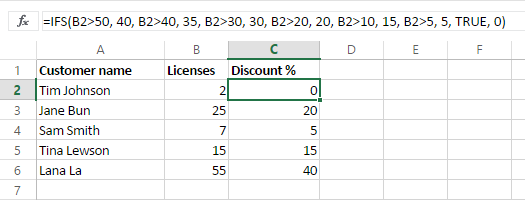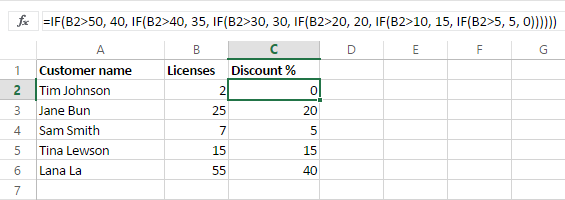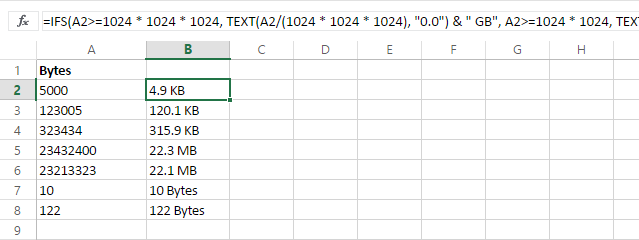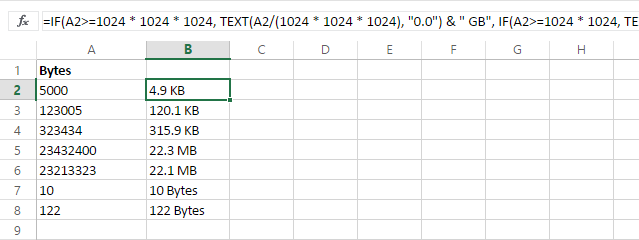# Use the new Excel IFS function instead of nested IF

From this short tutorial you'll learn about the new IFS function and see how it simplifies writing nested IF in Excel. You'll also find its syntax and a couple of use cases with examples.

Nested IF in Excel is commonly used when you want to evaluate situations that have more than two possible outcomes. A command created by nested IF would resemble "IF(IF(IF()))". However this old method can be challenging and time consuming at times.

The Excel team has recently introduced the IFS function that is likely to become your new favorite one. Excel IFS function is available only in Excel 365, Excel 2021 and Excel 2019.

### The Excel IFS function - description and syntax

The IFS function in Excel shows whether one or more conditions are observed and returns a value that meets the first TRUE condition. IFS is an alternative of Excel multiple IF statements and it is much easier to read in case of several conditions.

Here's how the function looks like:

IFS(logical_test1, value_if_true1, [logical_test2, value_if_true2]…)

It has 2 required and 2 optional arguments.

• logical_test1 is the required argument. It's the condition that evaluates to TRUE or FALSE.
• value_if_true1 is the second required argument that shows the result to be returned if logical_test1 evaluates to TRUE. It can be empty, if necessary.
• logical_test2…logical_test127 is an optional condition that evaluates to TRUE or FALSE.
• value_if_true2…value_if_true127 is an optional argument for the result to be returned if logical_testN evaluates to TRUE. Each value_if_trueN relates to a condition logical_testN. It can also be empty.

Excel IFS lets you evaluate up to 127 different conditions. If a logical_test argument doesn't have certain value_if_true, the function displays the message "You've entered too few arguments for this function". If a logical_test argument is evaluated and corresponds to a value other than TRUE or FALSE, IFS in Excel returns the #VALUE! error. With no TRUE conditions found, it shows #N/A.

### The IFS function vs. nested IF in Excel with use cases

The benefit of using the new Excel IFS is that you can enter a series of conditions in a single function. Each condition is followed by the result that will be used if the condition is true making it straightforward to write and read the formula.

Let's say you want to get the discount according to the number of licenses the user already has. Using the IFS function, it will be something like this:

`=IFS(B2>50, 40, B2>40, 35, B2>30, 30, B2>20, 20, B2>10, 15, B2>5, 5, TRUE, 0)`Here's how it looks with nested IF in Excel:

`=IF(B2>50, 40, IF(B2>40, 35, IF(B2>30, 30, IF(B2>20, 20, IF(B2>10, 15, IF(B2>5, 5, 0))))))`The IFS function below is easier to write and update than its Excel multiple IF equivalent.

`=IFS(A2>=1024 * 1024 * 1024, TEXT(A2/(1024 * 1024 * 1024), "0.0") & " GB", A2>=1024 * 1024, TEXT(A2/(1024 * 1024), "0.0") & " Mb", A2>=1024, TEXT(A2/1024, "0.0") & " Kb", TRUE, TEXT(A2, "0") & " bytes")``=IF(A2>=1024 * 1024 * 1024, TEXT(A2/(1024 * 1024 * 1024), "0.0") & " GB", IF(A2>=1024 * 1024, TEXT(A2/(1024 * 1024), "0.0") & " Mb", IF(A2>=1024, TEXT(A2/1024, "0.0") & " Kb", TEXT(A2, "0") & " bytes")))`## You may also be interested in

### 37 comments to "Use the new Excel IFS function instead of nested IF"

1.Fernanda says:

Hello,

I'm trying to get the following formula to work but this message keeps showing: "You've entered too few arguments for this function."

The formula in question: *the excel software is programmed to be in french so SI.CONDITIONS translates to IFS*

=SI.CONDITIONS(C20="Anglais 1"; 134-104; C20="Anglais 2"; 134-204; C20="Anglais 3"; 134-304; C20="Anglais 4"; 134-404; C20="Anglais 5"; 134-504; C20="Arts Plastiques 4"; 168-402; C20="Arts Plastiques 5"; 168-502; C20="Chimie 5"; 051-504; C20="Éducation Financière"; 102-522; C20="Espagnol"; 141-514;"0")

I know in this article, it states that if that specific message appears it is because I never put a value_if_true. But I think I did, but now I'm not too sure. Is it because my values have a dash ?

•Alexander Trifuntov (Ablebits Team) says:

Hello!
Use double quotes. Instead of 134-104, write "134-104" and so on.

•Fernanda says:

Hello,

I tried using the double quotes as you suggested and the same message keeps appearing. Should I consider doing another formula ?

•Fernanda says:

IT WORKED !! I'm so happy haha Turns out that I had to eliminate the "0" value at the end and replace it with the end of my parenthesis. Thank you so much for your help !!

2.Charne says:

Hi, I want to build a couple of formulas for current and previous month scores based on a table of data. Basically, if today's date is in the month of x (shown in column A), then display a specific score from column B.

In A2:A13, I have the names of the month's of the year.
In B2:B13, I have scores that are totals for that given month.

In D1, I want to display the current month's score based on today's date.
In D2, I want to display the previous month's score based on today's date.

I know it involved an IFS statement using TODAY(), and the appropriate cell in column B not being blank, and I'm at a loss on how to put it all together.

•Alexander Trifuntov (Ablebits Team) says:

Hello!
If I understand your task correctly, the following formula should work for you:

=SUMIF(A2:A13,TEXT(TODAY(),"mmmm"),B2:B13)

Hope this is what you need.

•Charne says:

Thanks Alexander, very close.

I'm looking to show the specific score for the corresponding month, not the sum of the scores.

So if A11's value is October, and Todays date is October 31st, show the value in B11

•Alexander Trifuntov (Ablebits Team) says:

Hello!
Have you tried using a formula? If your data contains only one cell "October", then you will get the score for October.
You can also use the VLOOKUP function to find the value:

=VLOOKUP(TEXT(TODAY(),"mmmm"),A2:B13,2,FALSE)

3.Rachel says:

Hi, I hope you can help me. I am looking for a formula that will calculate the date range between cells, (NETWORKDAYS, no holidays), where the cells used in the date range will depend if the are populated.

The calculation needs to consider the following:

C1 is the end date, and the start date is either B1 or A1.

B1 is the first start date to look at. If B1 is populated, then calculate the "networkdays" between B1 & C1.

If B1 is blank, then calculate the "networkdays" between A1 & C1.

If C1 is blank, the cell should be blank, even if A1 and B1 are populated.

This is what I have, and it works until C1 is blank:

=IF(B1="",NETWORKDAYS(A1,C1),NETWORKDAYS(B1,C1)))

•Alexander Trifuntov (Ablebits Team) says:

Hello!
If I understand correctly, add another nested condition to the IF formula:

=IF(C1<>"",IF(B1="",NETWORKDAYS(A1,C1),NETWORKDAYS(B1,C1)),"")

•Rachel says:

Thank you very much, Alexander, it works (with the addition one an extra closed parenthesis).

4.Aga says:

Hi, I'm trying to write formula that will return "BH" if cell contains "Brighton" and "LDN" if cell contains "London".
It woks only for cells wit "Brighton", but not cells with London and I'm getting #VALUE! error.

=IFS(SEARCH("Brighton",C48691),"BH",SEARCH("London",C48691),"LDN")

Thank you.
Aga

•Alexander Trifuntov (Ablebits Team) says:

Hello!
Use the ISNUMBER function to prevent errors from occurring.

=IFS(ISNUMBER(SEARCH(“Brighton”,C48691)),”BH”,ISNUMBER(SEARCH(“London”,C48691)),”LDN”)

•Aga says:

Thank you. I tried your formula, but now I'm getting #N/A error in both cases

•Alexander Trifuntov (Ablebits Team) says:

Hi!
Check the quotes in your formula. Change ” to "

•Aga says:

Hi!

I didn't notice it. Thank you very much for your help. That's great.

5.Sam says:

So the error was I didn't properly research how to formulate looking for "TRUE" statements. The "TRUE" in the formulas need to be without quotes.

6.Sam says:

Hello all,

I have a very long IFS() statement that I'm trying to get to work for a personal project to randomly generate a first name given three different criteria (all three of which are also randomly generated).

It's always returns the #N/A error, which I know is because it finds no "TRUE" statements. I've confirmed this by looking at both "Show Calculation Steps" and "Evaluate Formula" which shows the formula is properly randomly generating a name from each column. But they are all preceded by "FALSE," telling me that it's not recognizing any of the randomly generated criteria in the first place.

Here is an example of the recurring arguments I've put in to account for every outcome:
IFS(...,AND(AA22="Roman",X22="*",Z22="Female")="TRUE",VLOOKUP(RANDBETWEEN(1,50),RaceFirstName[#All],53),...)
AA=First Name's Origin [=VLOOKUP(RANDBETWEEN(1,100),RaceFirstName[#All],3)]
X=Race [=VLOOKUP(RANDBETWEEN(1,100),RaceFirstName[#All],2)]
Z=Gender [=VLOOKUP(RANDBETWEEN(1,2),Gender[#All],2)]
The VLOOKUP is looking at a large table and randomly selecting a name from there.

I've gone through the "RaceFirstName" and "Gender" tables to make sure there was no space at the end or beginning (as that's an issue I've found to be common).

The only three issues I can think of is
1. that the colums X, Z, and AA are still viewed as formulas and not values by the IFS() statement.
2. that the order of the references need to be X, Z, AA and not as they are now (currently not in that order)
3. that the formula itself has too many conditions (it has about 60 conditions, but maybe due to the AND statements, it's too much for it to want to calculate)

Any help or insight would be most welcome. Hopefully I was clear enough.

Thank you so much for taking the time to read this.

7.Walter says:

Thank you for taking the time to read this.

I would like to use IF to update a value in another field (that currently has a value). I am not sure how to enter just the current time.
=if(c2="x",b1=Now)

Ideally, someone makes c2 = x and then this happens. I don't know how to run a formula in the current field without overwriting the formula.

Thanks

•Alexander Trifuntov (Ablebits Team) says:

Hello!
If a value is written in a cell, then it is impossible to change it using a standard Excel formula. You need to use a VBA macro.

8.David Basham says:

I'm trying to write a formula that will analyze whether win, lose or tie a golf whole. I needs to consider the difference in player handicaps (me 2, competitor 11), the rating of the hole (7) and the 2 scores made on the whole (me 4, him 5), then return a -1 for a loss, a 0 for a tie or a +1 for a win. Example, I have to give my opponent 9 strokes, the distribution of which is determined by the rating of each hole. Hole 1 is rated #7 so he gets a stroke there. If I score 4 and he scores 5, we tie because 5-1 = 4. If I make 4 and he makes 6, I win because 6-1=5 which is higher than 4. If we both score 4, he wins because his 4-1 beats my 4. How can I do this?

9.Muckth Dograa says:

Hi, I need Excel function for Calculating a certain % of Discount basis number of days from a date:
If Cut off Date is Greater than the Month indicated then 0%; If cut off date is Lower than the month indicated then return Value as per discount.
Cut off Date 1Apr 1May 1Jun 1Jul 1Aug 1Sep 1Oct 1Nov 1Dec 1Jan 1Feb 1Mar
A - 1st Feb 2020
B - 15th March 2020
C - 1st September 2020
D - 15th October 2020

•Alexander Trifuntov (Ablebits Team) says:

Hello!

=IFS(A1>DATE(2021,3,1),1,A1>DATE(2021,1,1),2,A1>DATE(2020,12,1),3,A1>DATE(2020,11,1),4,A1>DATE(2020,10,1),5)

In the first condition, use the farthest date.

10.Dharmendra Rana says:

Salab Calls form-To Incentive per calls
Slab1 1841-2084 2.50₹
Slab2 2025-2116 3.50₹
Slab3 2117-2207 4.50₹
Slab4 2208-2300 5.50₹
Slab5 2301-Above 10₹ how to calculate in total rupees by Excel formula.

11.Cat M Bourque says:

Every 2 weeks a payment is made and entered, the beginning amount of payment are decreased by 1 every second Thursday. During the off weeks an extra payment can be made and is entered in a separate Col. Col 3. When the extra payment is made i would like the reflection of that payment shown in Col 2 by subtracting from the number of payments remaining and not effecting the bi-weekly payment / remaining payments.

Payments remaining = 110

Col. 1 has a payment of \$200.00
Col. 2 has 109 (number of payments remaining)
with an IF statement,

-if(col. 1>0,payments remaining-1,"")

Note that each payment made the IF statement changes from Payments remaining-1 to Col. 2 (previous cell number) -1 so it would be

-if(col. 1>0,Previous cell-1,"") Previous cell being 109

In addition to this there is another Col, Col. 3 for EXTRA payments where, when entered on a row with no other payments entered, i would like THAT payment reflected in the payments remaining total.

If you want the spreadsheet let me know .... can email it to you

Thanks
Cat

12.Abdu says:

Hi everybody...
I have problem related, I think, to using IF function or IFS.
I have a Pivot filter including 3 options, means I can select 1 or All or Multiple product categories. I am using SUMIFS to extract the data from Excel table, and filter using Pivot filter. all working fine until I select 2 product categories, I eliminate the problem when selecting (ALL), but could not do that when I select 2 product categories, the result was all zero values. here is my function:

=SUMIFS(SalesData[revenue];SalesData[year];I\$4;SalesData[region];\$H5;SalesData[Category];IF(\$I\$2="(All)";"*";\$I\$2))

my attempt was nested IF:
IF(\$I\$2="(All)";"*";IF(\$I\$2="(Multiple Items)";\$I\$2))
but it dose not work, any help...

13.Mike De Butts says:

with nested IF(), latter redundant calculations are ignored (not calculated)

=IF(1=1,"Answer","this bit could be processor heavy but is ignored")

but using the 'evaluate formula' it would appear that IFS() works out all the bits of the formula, even if the first condition is met and therefore is redundant

=IFS(1=1,"answer", other condition IS verified, redundant terms all calculated)

Is this the case?

essentially, IFS does indeed look simpler to untangle, BUT is it actually getting excel to do a less efficient amount of calculating?

•Gennady Terekhov (Ablebits Team) says:

Hello, Mike,

We haven’t investigated the efficiency of the IF() and IFS() functions in all configurations.
Most likely, they work in the same way. For example, if you create two formulas like the ones below:
=IF(1=1,"1",IF("=1","2"))
=IFS(1=1,"1", "=1", "2")
They return the same result equal to «1».
But following your hypothesis, the =IFS(1=1,"1", "=1", "2") formula should have returned a #VALUE! error as the second condition in the formulas is wrong.

•Brady says:

Mike you are correct. It appears as though IFS is horribly inefficient compared to traditional nested IFS statements. Much easier to write but you end up sitting around longer waiting on calculations to finish.

14.Mahmood says:

hi
why in my excel(2010 version) "IFS" function does not work??
what should I do??

•Charlie says:

15.Fred says:

16.Renat Tlebaldziyeu (Ablebits Team) says:

Hi Faye,

You need to add the additional column in your table and use the following formula to increase the price by 5%:
=D1*1.05
Then please copy the added column and use the Paste Special - Values option to replace the values in Column D.

17.Faye says:

I need excel function to calculate and update old selling price of same product number located in different rows in same column
example:

Product#1001-099
Old Price = \$20.00
New Price should be increased by 5%

Product is in Column A
Selling Price is Column D

How do I increase and replace the old Selling Price
for this product that is in Column A,
Row 8, Row 101, Row228?

•Mikey says:
•Maria Azbel (Ablebits Team) says: